### 贪心算法 —— Leetcode系列

• 本文章系列为笔者leetcode刷题的记录、复习与分享，有错误的地方欢迎指正。

# 分配问题

## 分发饼干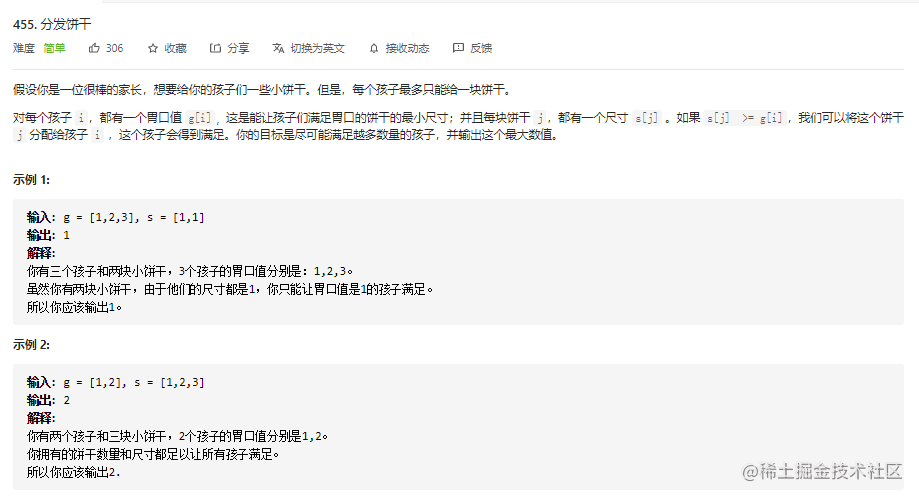`思路： 把孩子和饼干排序，给最小饥饿度的孩子分配最小的能饱腹的饼干。`

``````class Solution {
public:
int findContentChildren(vector<int>& g, vector<int>& s) {
int child = 0;
int cookies = 0;
sort(g.begin(),g.end());
sort(s.begin(),s.end());
while(child < g.size() && cookies < s.size()){
if(g[child]<=s[cookies]) child++;
cookies++;
}
return child;
}
};

## 分发糖果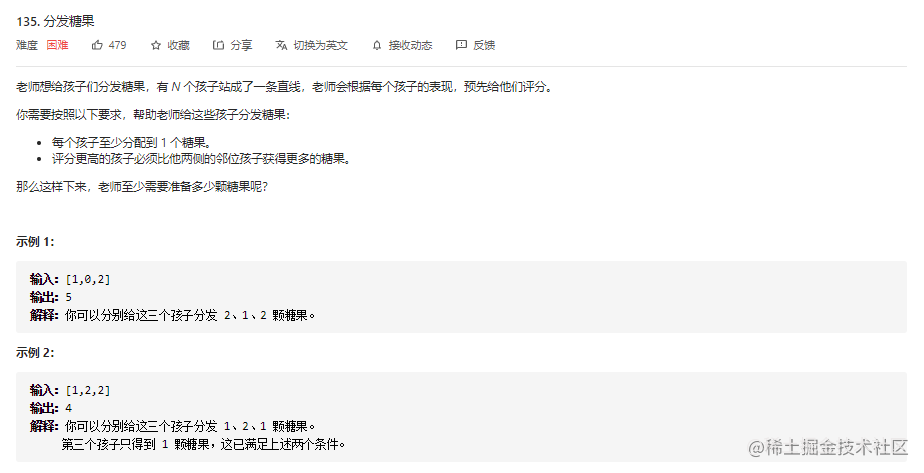``````class Solution {
public:
int candy(vector<int>& ratings) {
int size = ratings.size();
if(size<2) return size;
vector<int> candy(size,1);
for(int i =0;i <size-1;i++){
if(ratings[i+1]>ratings[i]){
candy[i+1] = candy[i]+1;
}
}
for(int i = size -1;i>0;i--){
if(ratings[i-1]>ratings[i]){
candy[i-1] = max(candy[i-1],candy[i]+1);
}
}
return accumulate(candy.begin(),candy.end(),0);
}
};

## 种花问题

• 自己为空
• 左边为空 或者 自己是最左
• 右边为空 或者 自己是最右

``````class Solution {
public:
bool canPlaceFlowers(vector<int>& flowerbed, int n) {
int s = flowerbed.size();
for(int i =0;i<s;i++){
if(flowerbed[i] ==0 && (i==0 || flowerbed[i-1]==0 ) && (i==s-1 || flowerbed[i+1]==0)){
n--;
if(n<=0) return true;
flowerbed[i] =1;
}
}
return n<=0;
}
};

# 区间问题

## 无重叠区间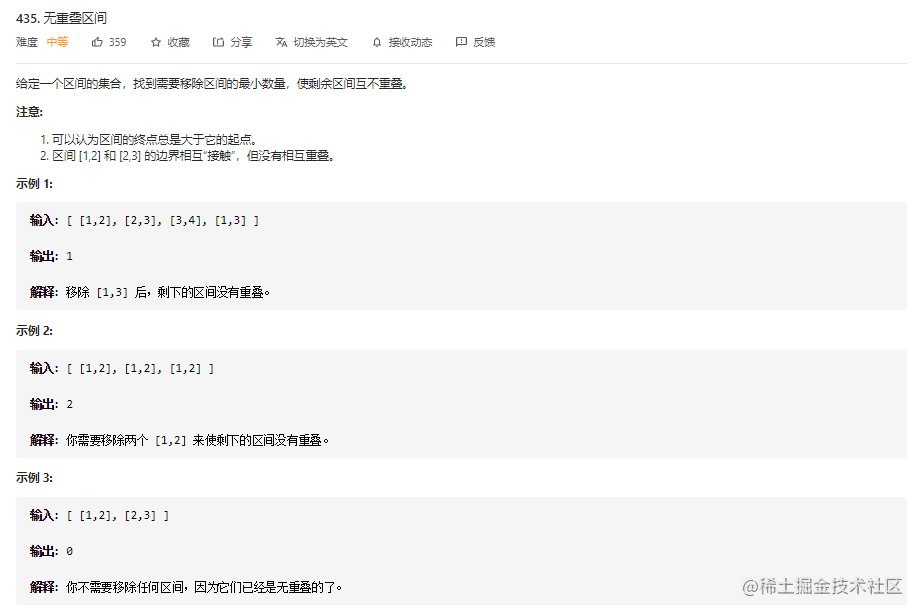思路: `先把区间按照结尾的大小进行增序排序，每次选择结尾最小且和前一个选择的区间不重叠的区间`

``````class Solution {
public:
int eraseOverlapIntervals(vector<vector<int>>& intervals) {
if(intervals.empty()) return 0;
int n = intervals.size();
if(n<2) return 0;
sort(intervals.begin(),intervals.end(), [](vector<int> a,vector<int>b){
return a<b;
});
int count =0,prev = intervals;
for(int i = 1;i<n;i++){
if(intervals[i]<prev){
count++;
} else {
prev = intervals[i];
}
}
return count;
}
};

## 用最少数量的箭引爆气球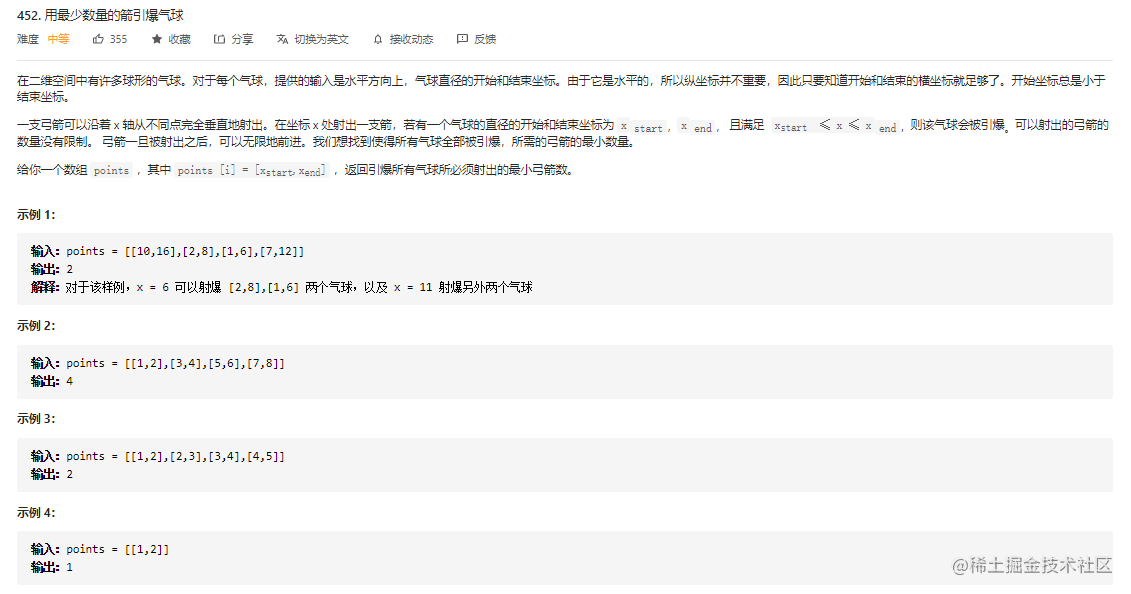• 区间结尾排序
• 拿当前区间的右端作为标杆（在保证当面区间被射到的前提下，尽可能找多的重合区间）。
• 只要下一个区间的左端<=标杆下一个区间的左端<=标杆，则重合，继续寻求与下一个区间重合
• 直到遇到不重合的区间，弓箭数+1
• 拿新区间的右端作为标杆，重复以上步骤

``````class Solution {
public:
int findMinArrowShots(vector<vector<int>>& points) {
int n = points.size();
if(n<=1) return n;
sort(points.begin(), points.end(), [](vector<int> A, vector<int> B){
return A<B;//不可以用开头排序，因为不能确保包含的区间是否重合（如[0,9],[0,6],[7,8]）
});
int cur = points,count = 1;
for(int i = 1; i<n;i++){
if(points[i]>cur){
count++;
cur = points[i];
}
}
return count;
}
};

## 划分字母区间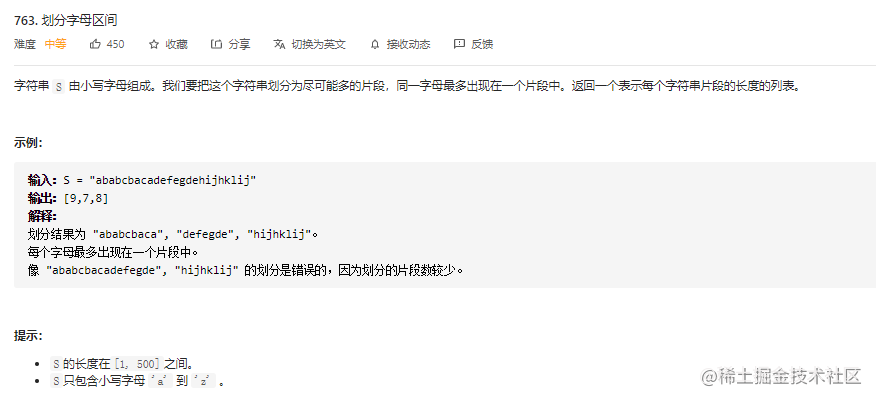思路：`由于同一个字母只能出现在同一个片段，显然同一个字母的第一次出现的下标位置和最后一次出现的下标位置必须出现在同一个片段。因此需要遍历字符串，得到每个字母最后一次出现的下标位置` 在得到每个字母最后一次出现的下标位置之后，可以使用贪心的方法将字符串划分为尽可能多的片段，具体做法如下。

• 从左到右遍历字符串，遍历的同时维护当前片段的开始下标`start`和结束下标`end`，初始时`start=end=0`

• 对于每个访问到的字母，得到当前字母的最后一次出现的下标位置，令`end=max(end,end1)`

• 当访问到下标end 时，当前片段访问结束，当前片段的长度为`end−start+1`，将当前片段的长度添加到返回值，然后令start=end+1，继续寻找下一个片段。

``````class Solution {
public:
vector<int> partitionLabels(string S) {
int map = {-1};
vector<int> res;
int star = 0, end = 0;
for(int i = 0; i < S.size(); i++) {
map[S[i] - 'a'] = i;
}
for(int i = 0; i < S.size();i++){
end = max(end, map[S[i] - 'a']);
if(i == end){
res.push_back(end-star+1);
star = i+1;
}
}
return res;
}
};449